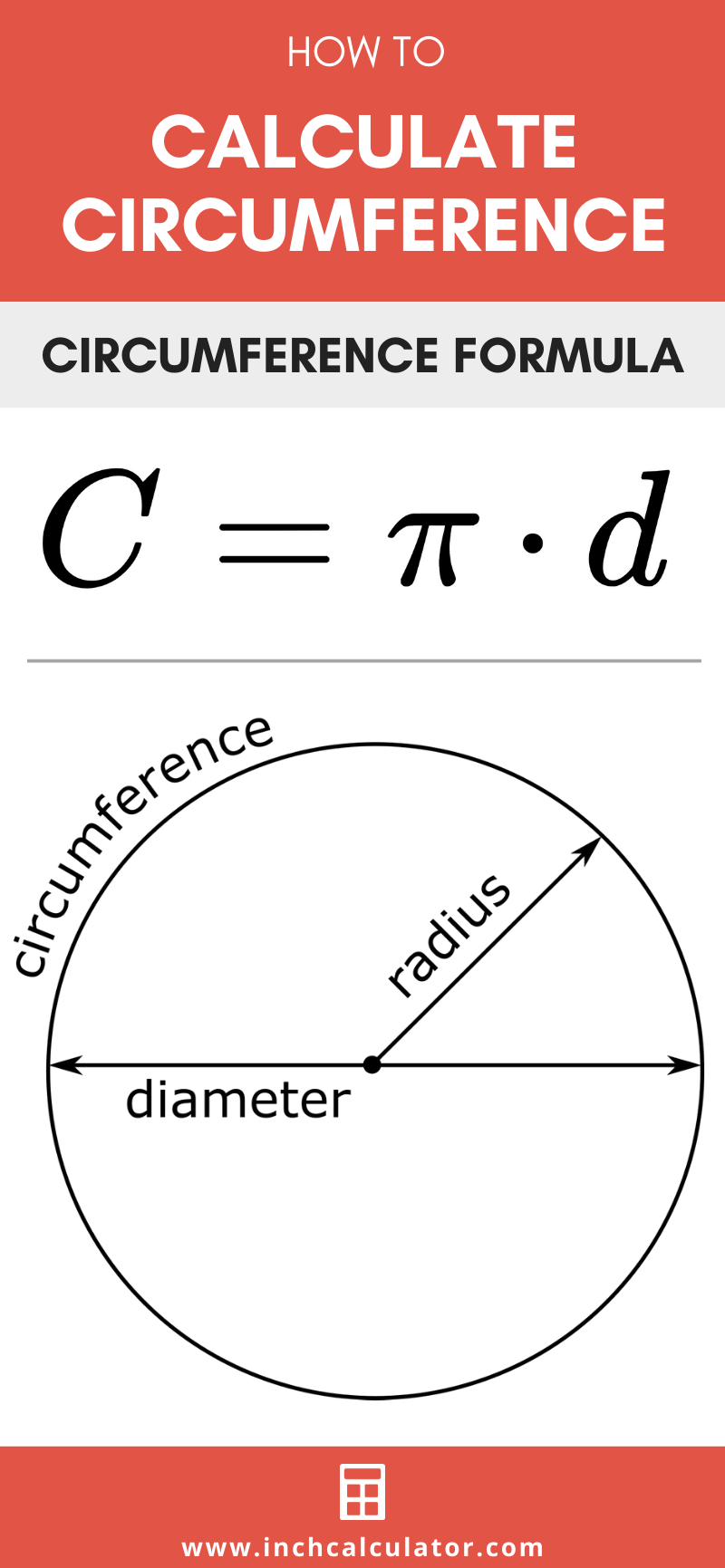# Circumference Calculator

Calculate the circumference of a circle using the radius, diameter, or area with the calculator below.

## How to Find the Circumference of a Circle

The circumference is the distance around a circle, also known as the perimeter of a circle. If the path around the circle were flattened out into a straight line, the line’s length would be the circumference.

### Circumference Formulas

So “how do you go about solving circumference?” you might ask. Use these formulas to solve.

#### Given the Radius

Given the radius of a circle, the formula for circumference is:

C = 2πr

The circumference C is equal to 2 times pi times the radius of the circle r.

#### Given the Diameter

Given the diameter of a circle, the formula for circumference is:

C = πd

The circumference C is equal to pi times the diameter of the circle d.

#### Given the Area

Given the area of a circle, the formula for circumference is:

C = 2 × π × A ÷ π

The circumference C is equal to 2 times pi times the square root of the area of the circle A divided by pi.

## How to Calculate the Diameter using the Circumference

It is possible to solve the diameter of a circle using the circumference. Since the diameter is equal to double the radius, use the inverse of the circumference formula to solve for the diameter.

d = C / π

Thus, the diameter d of a circle is equal to the circumference C divided by pi.

You might also be interested in our circle calculator for solving the other dimensions of a circle, including the radius, diameter, and area.